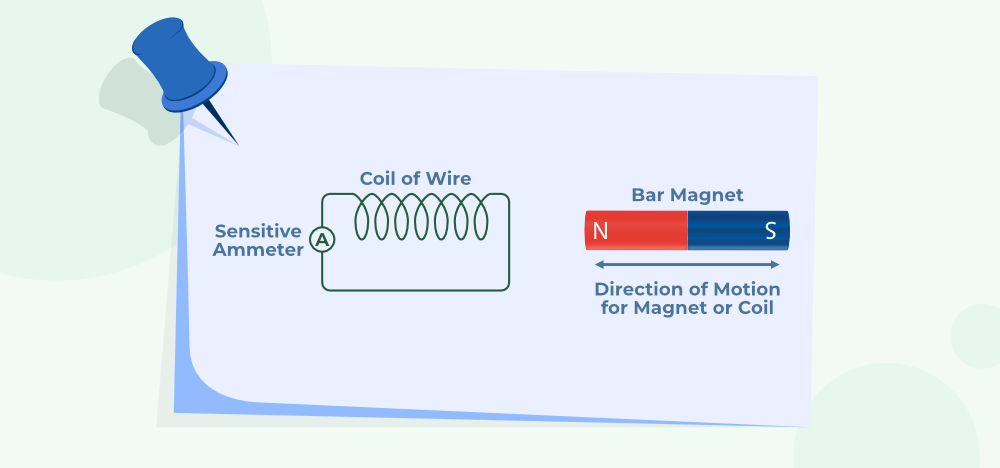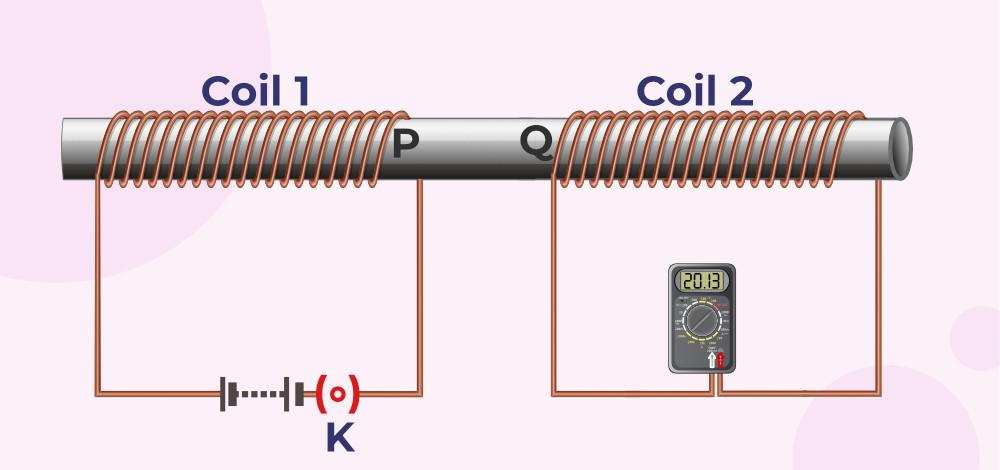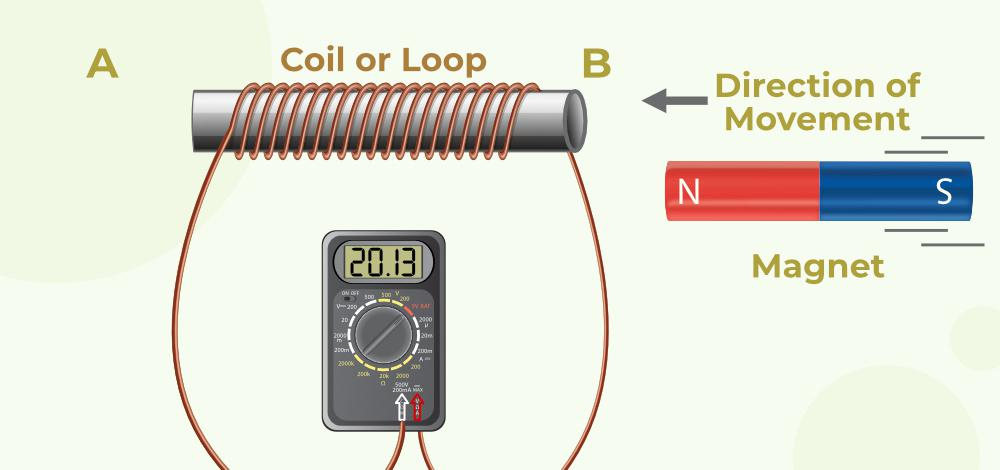GFG App
Open AppBrowser
Continue

# Faraday’s Laws of Electromagnetic Induction

Faraday’s Law of Electromagnetic Induction was given by an English scientist Michael Faraday. This law was proposed by Michael Faraday in 1831. According to Faraday’s Law of Electromagnetic Induction, the induced current in the circuit is directly proportional to the rate of change of magnetic flux.

## Faraday’s Laws of Electromagnetic Induction

Faraday has provided two laws that are the basis of modern electromagnetism that is,

• Faraday’s First Law of Electromagnetic Induction
• Faraday’s Second Law of Electromagnetic Induction

### Faraday’s First Law of Electromagnetic Induction

According to Faraday’s First Law of Electromagnetic Induction, “When the magnetic flux linked with closed-circuit changes, an emf is induced in it which lasts only as long as the change in flux is taking place. If the circuit is closed then current also gets induced inside the circuit which is called Induced current” Changing the magnetic field changes the induced current in the circuit.

The image given below shows the deflection in the coil according to the law of Electromagnetic Induction.Magnetic Fields Can be changed by,

• Moving a bar magnet towards or away from the coil.
• Moving the coil into the magnetic field or outside the magnetic field.
• Rotating the coil relative to the magnet.
• Changing the area of a coil placed in the magnetic field.

### Faraday’s Second Law of Electromagnetic Induction

According to Faraday’s Second Law of Electromagnetic Induction, “The magnitude of the induced emf is equal to the rate of change of magnetic flux linked with the coil.”

E = dⲫ/dt

E = -N dⲫ/dt

E = -N (ⲫ2-ⲫ1)/t

where,
E is Electromotive Force
N is the number of turns of the coil.
is the flux change

## Lenz’s law

Lenz’s is named after the German physicist “Emil Lenz “, who formulated it in 1834. According to Lenz “the direction of induced current in a circuit is such that it opposes the change in magnetic flux produced.” It is a scientific law that specifies the direction of induced current but states nothing about its magnitude.

According to Faraday’s Second Law of Electromagnetic Induction,

E = -N(d∅/dt)

Here, the negative sign indicates that the direction of induced emf is such that it opposes the change in magnetic flux which is in accordance with Lenz’s law

Faraday has performed three experiments that form the basis of electromagnetic induction.

### Experiment 1

In this experiment, Faraday took a circular coil and connected it with a galvanometer and now he takes a strong bar magnet. When the north pole of the bar magnet is moved towards the coil, the galvanometer showed deflection to the right side of the zero mark in the galvanometer. When the magnet is moved away from the coil again it showed deflection but in the opposite direction. Similarly, the experiment is done with the south pole of the bar magnet, again the deflection is observed but opposite to the direction shown by the north pole of the bar magnet. When the magnet is held stationary near the coil, no deflection is observed in the galvanometer.

Conclusion: As the magnet is moved closer to the coil the magnetic flux increases hence, an induced current setup in the coil in one direction. When the magnet is moved away from the coil, the magnetic flux decreases, hence an induced current set up in the coil in the opposite direction. When the magnet is held stationary near the coil, there is no change in the magnetic flux.

### Experiment 2

In this experiment bar magnet is kept stationary and the coil is moved. The same result is observed in experiment 1. When the relative motion between the magnet and coil is fast, the deflection in the galvanometer is larger and vice versa.

### Experiment 3

As you can see from the figure below. Two coils primary (p) and secondary (s), are wound on cylindrical support. The primary coil is connected to a key, a rheostat, and a battery. The secondary is connected with a galvanometer. When the key is pressed in the primary coil the galvanometer shows deflection in one direction. When the key is released, it again shows deflection but in the opposite direction. When the key is kept pressed steady current flows through the primary coils, and the galvanometer does not show any deflection. When the current in the primary coil is increased with the help of the rheostat, the induced current flows in the secondary coil in the same direction as that of the primary coil.

The image given below shows the setup of Faraday’s Experiment.All three Faraday Experiments is can be summarised in the table given below,

Now we take a magnet approaching a coil and consider instances at times T1 and T2

At time T1 flux linked with the coil = NΦ1

At time T2 flux linked with the coil = NΦ2

Change in flux = N(Φ2 – Φ1)

Rate of change of flux = N(Φ2 – Φ1) / t

Taking the derivative of the above equation, we get

N dΦ/dt

Faraday’s second law of electromagnetic induction, says that the induced emf in a coil is equal to the rate of change of flux associated with the coil. Thus,

E = – N dΦ/dt…(1)

The negative sign is added as it helps to accommodate Lenz’s law.

### Change in Electromagnetic Force

Electromagnetic force linked with the coil can easily be changed by following the steps discussed below.

• Induced emf can easily be increased by increasing the number of turns in the coil.
• If the magnetic field strength increases induced emf also increases

The image given below tells us about the change in electromagnetic force linked with the coil when the above conditions are met.Faraday’s law has various applications and some of the common applications of Faraday’s Law are,

• Faraday’s Law is used in electrical equipment like transformers and electric motors.
• Induction cooker works on the principle of mutual induction, which is derived from Faraday’s law.
• Faraday’s law is also helpful in designing musical instruments like the electric guitar, electric violin, and others.

## Solved Examples on Faraday’s Law of Electromagnetic Induction

Example 1: The magnetic flux linked with a coil is changed from 2Wb to 0.2Wb in 0.5 seconds. Calculate the induced emf.

Solution:

Δⲫ = 0.2-2 = 1.8wb

Δt = 0.5 sec

E = -(Δⲫ/Δt)

E= -1.8/0.5 volts

E= -3.6 volts

Therefore, induced emf will be -3.6 volts.

Example 2: In a coil of resistance 200, a current is induced by changing the magnetic flux through it as shown in the figure. Calculate the magnitude of change in flux through the coil.

Solution:

dq = – (N/R) dt

i = (1/R). (dq/dt)

Δⲫ = R.Δq

Δⲫ = 200 × (Area of circular graph)

Δⲫ = 200 × (1/2×20×0.5)

Δⲫ = 200 × 5

Δⲫ = 1000 Wb

Therefore, magnitude of change in flux is 1000 Wb.

Example 3: Calculate the emf induced in the wire. When a small piece of metal wire dragged across the gap between the pole pieces of a magnet in 0.6sec. The magnetic flux between the pole pieces is known to be 9×10-4 Wb.

Solution:

dt = 0.5 s

dⲫ = 9×10-4-0 = 9×10-4 Wb

E = (dⲫ)/dt

E= (9×10-4)/0.6

E= 0.0036 V

Therefore, the induced emf 0.0036V

## FAQs on Faraday’s Law of Electromagnetic Induction

### Question 1: State Faraday’s Law of Electromagnetic Induction.

There are two laws explained by Faraday called Faraday’s Laws of Electromagnetic Induction. First law explains the induction of emf in a conductor and the second law tells the emf produced in the conductor.

### Question 2: What is Faraday’s First Law of Electromagnetic Induction?

According to Faraday’s first law of Electromagnetic Induction, “ An EMF is produced when a conductor is placed in a varying magnetic field”, and the current produced in this process is called Induced Current.

### Question 3: What is Faraday’s Second Law of Electromagnetic Induction?

According to Faraday’s second law of Electromagnetic Induction, “ The rate of change of flux is directly proportional to the induced current in a coil.”

### Question 4: Why are Faraday’s Laws important?

Faraday’s law is used to define the EMF produce inside a coil if it rotates in a magnetic field. This concept is widely used in modern-day physics.

• It is used in electric motors.
• It is used in electric generators.